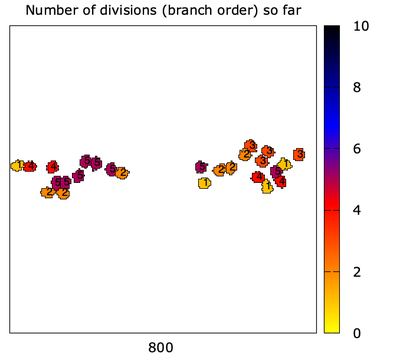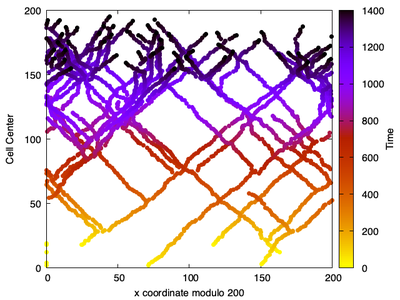# Correlated Random Walkers

##### Persistent Identifier

Use this permanent link to cite or share this Morpheus model:

Particles moving left and right (as well as up) to mimic actin filament barbed ends

## Introduction

Particles are moving left and right (as well as up) to mimic actin filament barbed ends as motivated by the referenced paper.

## Description

Initialize with a few close to $y = 0$, assign them random left or right directions.

Assymetric CellDivision is used to create branches at some constant probability. One daughter goes left, one goes right.

This simulation mimics the ‘unlimited Arp2/3 scenario’ where branching is not limited, and where branching takes place only close to the filament barbed ends.Snapshot of the actual locations of the barbed ends with the ‘branch order’ (i.e. number of divisions) that they belong to assigned to each of them.

The trajectory of the particles represents the actin filaments. The cell $x$ coordinate modulo the size of the $x$ range is also kept, so that the cell trajectories ‘wrap around’ (so we see ‘the filaments’ of ends that wandered off the edge of the domain).‘Trails’ left by the particles, i.e. the branched structure of the actin filaments.

## Reference

H. P. Grimm, A. B. Verkhovsky, A. Mogilner, J.-J. Meister: Analysis of actin dynamics at the leading edge of crawling cells: implications for the shape of keratocyte lamellipodia. Eur. Biophys. J. 32: 563–577, 2003.

## Model

Get this model via:

XML Preview

<MorpheusModel version="4">
<Description>
<Title>CRW-ActinBranchingUnlimited Arp2-3</Title>
<Details>"Correlated Random Walkers":
Particles moving left and right (as well as up) to mimic actin filament barbed ends as in the paper:
Grimm HP, Verkhovsky AB, Mogilner A, Meister JJ. Analysis of actin dynamics at the leading edge of crawling cells: implications for the shape of keratocyte lamellipodia. European Biophysics Journal. 2003 Oct 1;32(6):563-77.
Initialize with a few close to y=0, assign them random left or right directions
Assymetric "cell division" is used to create branches at some constant probability. One daughter goes left, one goes right.
This simulation mimics the "unlimited Arp2/3 scenario" where branching is not limited,
and where branching takes place only close to the filament barbed ends.
The trajectory of the particles represents the actin filaments.
The cell x coordinate modulo the size of the x range is also kept, so that the cell trajectories "wrap around"
(so we see "the filaments" of ends that wandered off the edge of the domain)
Code: Leah Edelstein-Keshet
Mathematical Models in Cell Biology
</Details>
</Description>
<Global>
<Constant value="0.0" symbol="xc"/>
</Global>
<Space>
<SpaceSymbol symbol="space"/>
<Lattice class="square">
<Size value="200, 200, 0" symbol="size"/>
<BoundaryConditions>
<Condition boundary="x" type="periodic"/>
<Condition boundary="y" type="noflux"/>
<Condition boundary="-y" type="noflux"/>
<Condition boundary="-x" type="periodic"/>
</BoundaryConditions>
<Neighborhood>
<Order>2</Order>
</Neighborhood>
</Lattice>
</Space>
<Time>
<StartTime value="0"/>
<StopTime value="1400"/>
<TimeSymbol symbol="time"/>
</Time>
<CellTypes>
<CellType name="medium" class="medium"/>
<CellType name="BarbedEnd" class="biological">
<Annotation>The barbed ends are assumed to grow left and right along a "motile cell edge" (not explicitly modeled). The actin filaments are depicted by the trails formed by barbed ends. Those are shown in the analysis, but not modeled explicitly otherwise.</Annotation>
<VolumeConstraint target="50" strength="1"/>
<ConnectivityConstraint/>
<Property value="if(rand_uni(0,1)&lt;0.5,1,-1)" name="the direction" symbol="s"/>
<Property value="0.0" name="Number of divisions (branch order) so far" symbol="nd"/>
<Property value="0.0" name="x coordinate modulo 200" symbol="xc"/>
<DirectedMotion strength="1.0" name="Move right/left (+1, -1) and up" direction="s, 1, 0.0"/>
<CellDeath name="some death to avoid too many ">
<Condition>rand_uni(0,1)&lt;0.0002</Condition>
<Annotation>The "death" rate represents capping of an actin filament that prevents a barbed end from growing further. Those ends are essentially "dead".</Annotation>
</CellDeath>
<CellDivision orientation="1.0, 0.0, 0.0" daughterID="daughter" division-plane="oriented" name="Assymetric division">
<Condition>rand_uni(0,1)&lt;0.002</Condition>
<Triggers name="Create one daughter going left, one going right">
<Rule symbol-ref="s" name="assign different direction to each daughter">
<Expression>if(daughter==1,-1,1)</Expression>
</Rule>
<Rule symbol-ref="nd" name="increase branch order by one">
<Expression>nd+1</Expression>
</Rule>
</Triggers>
<Annotation>The "division" represents an event where the actin filament branches (via the Arp2/3 complex). Here the branching is assumed to take place right at the barber end. Each branching results in one daughter branch going left and a second gowing right. This is implemented using the asymetric cell division of Morpheus.</Annotation>
</CellDivision>
<System name="x coordinate modulo 200" solver="Dormand-Prince [adaptive, O(5)]">
<Rule symbol-ref="xc" name="Compute x coordinate modulo size of x axis">
<Expression>mod(cell.center.x,size.x)</Expression>
</Rule>
<Annotation>This modified x-coordinate is used for the wrap-around domain, so that particles that leave the domain edge are  given a coordinate modulo the domain length. This method is used to insure that the trails (a.k.a. actin flaments) of all the particles are shown on the same periodic domain.</Annotation>
</System>
</CellType>
</CellTypes>
<CPM>
<Interaction default="0.0">
<Contact value="40" type2="BarbedEnd" type1="BarbedEnd"/>
<Contact value="20" type2="medium" type1="BarbedEnd"/>
</Interaction>
<MonteCarloSampler stepper="edgelist">
<MCSDuration value="1"/>
<Neighborhood>
<Order>2</Order>
</Neighborhood>
<MetropolisKinetics temperature="2"/>
</MonteCarloSampler>
<ShapeSurface scaling="norm">
<Neighborhood>
<Order>6</Order>
</Neighborhood>
</ShapeSurface>
</CPM>
<CellPopulations>
<Population size="1" type="BarbedEnd">
<InitRectangle mode="random" random-offset="5" number-of-cells="5">
<Dimensions size="200.0, 20.0, 0.0" origin="0.0, 0.0, 0.0"/>
</InitRectangle>
<Annotation>Initate all the barbed ends close to the lower part of the domain, so we can watch them move up.</Annotation>
</Population>
</CellPopulations>
<Analysis>
<Gnuplotter time-step="20" decorate="true">
<Terminal name="png"/>
<Annotation>Plot the actual locations of the barbed ends and assign them each the "branch order" (i.e. number of divisions) that they belong to.</Annotation>
<Plot>
<Cells flooding="true" value="nd" min="0">
<ColorMap>
<Color value="0" color="yellow"/>
<Color value="5" color="red"/>
<Color value="8" color="blue"/>
<Color value="12" color="black"/>
</ColorMap>
</Cells>
<CellLabels value="nd"/>
</Plot>
</Gnuplotter>
<Logger time-step="10">
<Annotation>Draw the "trails" left by the particles, i.e. the branched structure of the actin filaments.</Annotation>
<Input>
<Symbol symbol-ref="cell.center.x"/>
<Symbol symbol-ref="cell.center.y"/>
</Input>
<Output>
<TextOutput/>
</Output>
<Plots>
<Plot time-step="-1">
<Style style="points" point-size="1"/>
<Terminal terminal="png"/>
<X-axis maximum="200.0" minimum="0.0">
<Symbol symbol-ref="xc"/>
</X-axis>
<Y-axis maximum="200.0" minimum="0.0">
<Symbol symbol-ref="cell.center.y"/>
</Y-axis>
<Color-bar reverse-palette="true">
<Symbol symbol-ref="time"/>
</Color-bar>
</Plot>
</Plots>
</Logger>
<ModelGraph reduced="false" include-tags="#untagged" format="svg"/>
</Analysis>
</MorpheusModel>